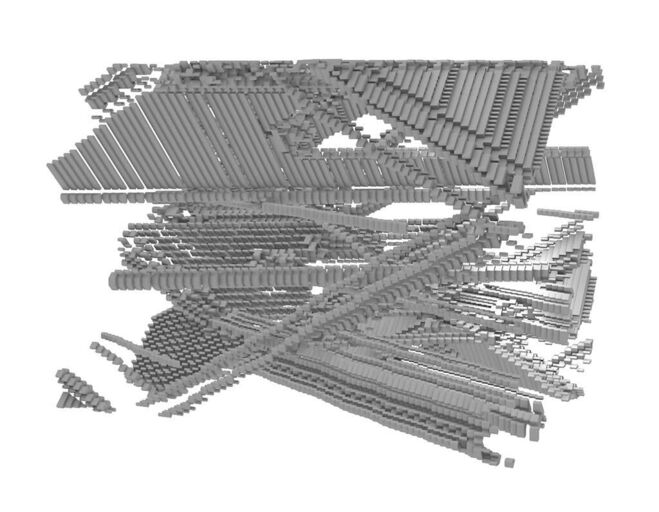# Sketch For A Modular Construction With Cellular AutomatonAuthors:
• Sina Seifee
Authors:
Year: 2011
Categories: Sculpture / Objects, model
Project type: Seminarprojekt
Cellular automata are simple mathematical principles. They consist of a lattice of discrete identical sites with each site taking on a finite set of values. The values of the sites evolve in discrete timesteps according to deterministic rules that specify the value of each site in terms of the values of its neighboring sites. Cellular automata may thus be considered as discrete idealizations of the partial differential equations often used to describe natural systems. Their discrete nature also allows an important analogy with digital computers: cellular automata may be viewed as parallel-processing computers of simple construction. One of the most fundamental properties of a cellular automaton is the type of grid on which it is computed. Cellular automata may also be constructed on Cartesian grids in arbitrary numbers of dimensions. (see: Cellular-Automata, Stephen Wolfram: Los Alamos Science, 9, 1983) The evolution of a two-dimensional cellular automaton can be made by starting with the initial state in the first row (generation zero), the first generation on the second row, and so on. This can be extended in three dimensions. By connecting the neighboring sites as lines in arbitrary selection rules, for example in perpendicular columns, we get closed shapes that, being put together construct a spatial model, serving the initial formula of cellular automata.
Collaboration:
Lab.D, MAKK, Urs Fries, Bernd Voss
Supervision:
Zilvinas Lilas
Authors:
• Sina Seifee
A production of the Academy of Media Arts Cologne.
External cooperation:
Museum für Angewandte Kunst Köln
Source:
Archiv Kunsthochschule für Medien Köln